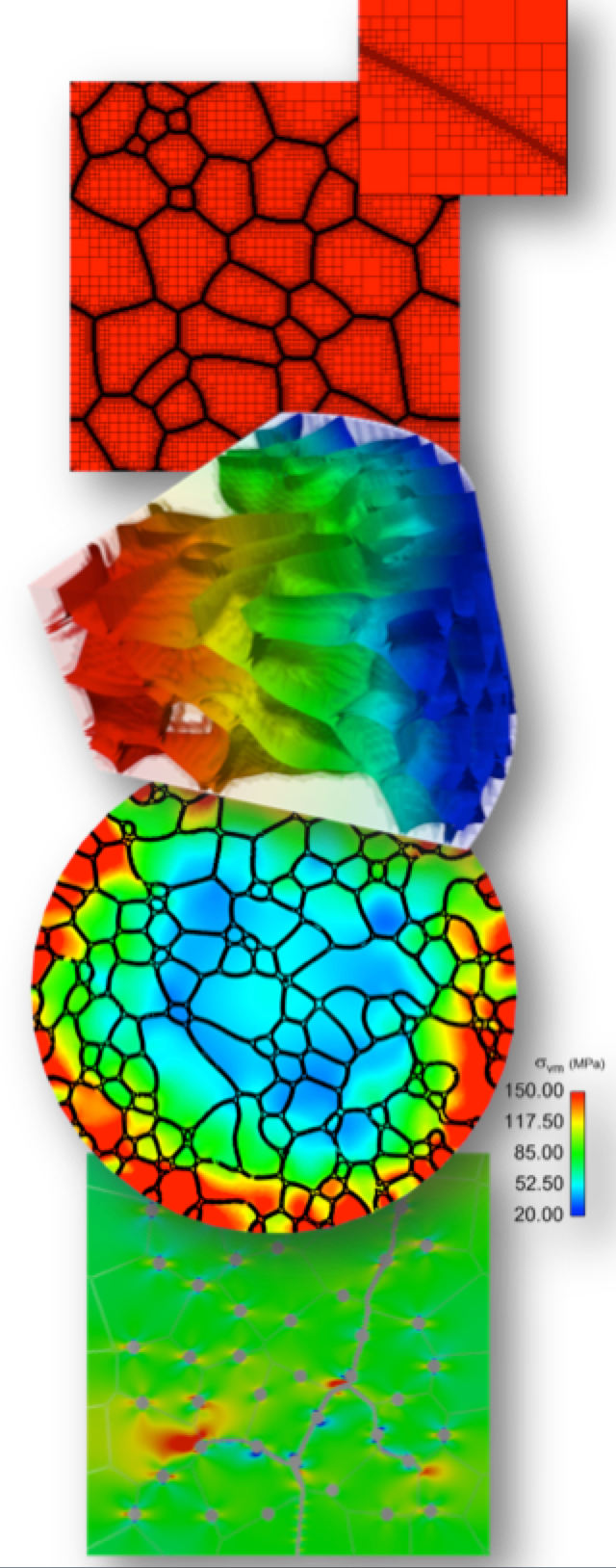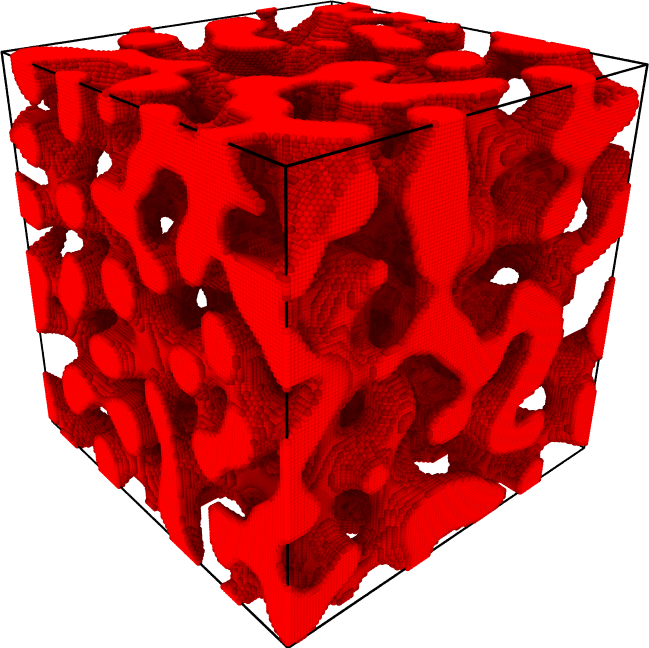# Phase Field ModulePhase field results produced using the MOOSE Phase Field Module

The MOOSE phase field module is a library for simplifying the implementation of simulation tools that employ the phase field model. Multiphysics capability that includes mechanics and heat conduction can be obtained by employing the tensor mechanics and heat conduction modules. More information about this module is found below:

## Basic Phase Field Model Information

• Basic Phase Field Equations: Basic information about the equations underlying the phase field module

• Expression Builder: Using automatic differentiation of free energy material objects

• Solving Phase Field Models: Basic info about solving phase field models

• Function Material Kernels: Working with Function Materials that carry around their own derivatives

• Phase Field Model Units: Discussion of units in phase field models

• Anisotropy: Support of anisotropic mobilities and interfacial energies

• CALPHAD: Using thermodynamic databases to parameterize phase field models

• Quantitative Two Component Polynomial Free Energies: Simple two component models using polynomial free energies

• FAQ: Frequently asked questions about the phase field modules

## Multiple Phase Models

MOOSE provides capabilities that enable the easy development of multiphase field model. A free energy expression has to be provided for each individual phase. Different systems exist to combine those _phase free energies_ into a _global free energy_.

• Two-phase Models: Two phases, one phase order parameter

• Kim-Kim-Suzuki Model: per-phase concentrations, two phases

• Multiphase Models: phases, phase order parameters

• Grand Potential Model: solving a Legendre transform of the phase field equations, where the independent variable is the chemical potentialAtomistic input file generated using the SolutionRasterizer.

## Multiphysics Coupling

• Mechanics Coupling - Coupling phase field equations with mechanics

## Phase field sub-systems

### Grain Growth

• Grain Growth Model: Background on the phase field model implemented in MOOSE

• Grain Boundary Anisotropy

• Elastic Driving Force For Grain Growth

## Initial Conditions

• Initial Conditions: Basic phase field initial conditions

• Image Reader: Reconstructing initial conditions from images (SEM, optical, etc.)

• EBSD Reader: Reconstructing initial conditions from EBSD and EDS data

# Tutorials

• Fe-Cr Phase Decomposition: Illustrates using parsed function kernels to create a two phase decomposition simulation